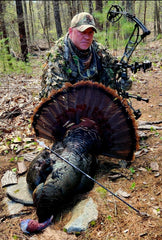DONALD FRANCIS  Spurs - 2x1" = 2 Beard - 10.5" = 11 Weight - 21.43lbs = 22.86 Total score = 35.86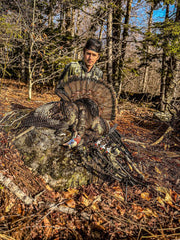COLE BOISVERT  Spurs - 2 x 3/4" = 1.50 Beard - 9" = 9 Weight - 20lbs = 20 Total score = 33.5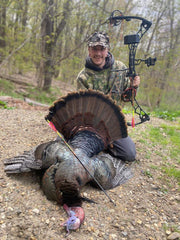BRET WITOWSKI  Spurs - 2 x 7/8" = 1.76 Beard - 9" = 9 Weight - 23.5lbs = 27 First Turkey = 2 Total score = 39.76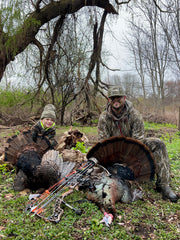TYLER FORBES  Spurs - 2 x 1" = 2 Beard - 9 5/16" = 9.32 Weight - 20lbs = 20 Filmed Hunt = 2 Total score = 33.32ASHTON HANNAH Spurs - 2 x 13/16" = 1.63 Beard - 8 1/4" = 8.25 Weight - 19.6lbs = 19.60 Kill Shot Film = 1 Total score = 30.48JOE CRAFTS (youth) Spurs - 2 x 7/8" = 1.75 Beard - 8 7/8" = 8.88 Weight - 21.41lbs = 22.82 Youth +2 Total score = 35.45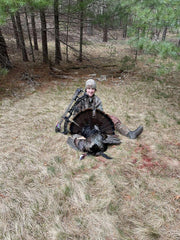COLE JENKINS (youth) Spurs - 2 x 1 1/8" = 2.5 Beard - 9 1/4" = 9.25 Weight - 20.1 lbs = 20.2 Kill shot on film +1  First Bird +2  Youth +2 Total score = 36.95